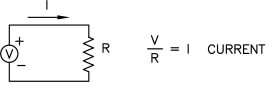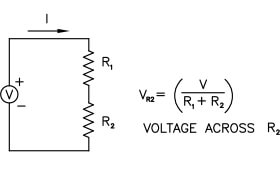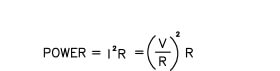Resistors are electrical devices that resist the flow of electricity. They can be used to

• 1. Limit current in an electrical circuit
• 2. Reduce voltage in portions of an electrical circuit
• 3. Dissipate electrical energy by turning it into heat (as with load resistors)

Power resistors are designed to handle larger amounts of power than typical resistors, generally more than 5 Watts. They are used in many types of industrial and test equipment, power supplies and amplifiers.

High voltage resistors are designed to handle higher voltages than typical resistors, generally more than 1000 Volts.

OhmWeve resistors are good at both and are also compact, lightweight and easy to mount in one of our OhmWeve resistor cages. They are woven with nichrome wire and high temperature yarn.

The circuit diagram below shows how a resistor is used to limit current in a circuit.This circuit diagram shows how a resistor is used to reduce voltage in a circuit.The following equation shows how to calculate the electrical power dissipated by a resistor.